# The MIXED Procedure

### Example 65.2 Repeated Measures

The following data are from Pothoff and Roy (1964) and consist of growth measurements for 11 girls and 16 boys at ages 8, 10, 12, and 14. Some of the observations are suspect (for example, the third observation for person 20); however, all of the data are used here for comparison purposes.

The analysis strategy employs a linear growth curve model for the boys and girls as well as a variance-covariance model that incorporates correlations for all of the observations arising from the same person. The data are assumed to be Gaussian, and their likelihood is maximized to estimate the model parameters. For overviews of this approach to repeated measures, see Jennrich and Schluchter (1986); Louis (1988); Crowder and Hand (1990); Diggle, Liang, and Zeger (1994); Everitt (1995). Jennrich and Schluchter present results for the Pothoff and Roy data from various covariance structures. The PROC MIXED statements to fit an unstructured variance matrix (their Model 2) are as follows:

data pr;
input Person Gender \$ y1 y2 y3 y4;
y=y1; Age=8;  output;
y=y2; Age=10; output;
y=y3; Age=12; output;
y=y4; Age=14; output;
drop y1-y4;
datalines;
1   F   21.0    20.0    21.5    23.0
2   F   21.0    21.5    24.0    25.5
3   F   20.5    24.0    24.5    26.0
4   F   23.5    24.5    25.0    26.5
5   F   21.5    23.0    22.5    23.5
6   F   20.0    21.0    21.0    22.5
7   F   21.5    22.5    23.0    25.0
8   F   23.0    23.0    23.5    24.0
9   F   20.0    21.0    22.0    21.5
10   F   16.5    19.0    19.0    19.5
11   F   24.5    25.0    28.0    28.0
12   M   26.0    25.0    29.0    31.0
13   M   21.5    22.5    23.0    26.5
14   M   23.0    22.5    24.0    27.5
15   M   25.5    27.5    26.5    27.0
16   M   20.0    23.5    22.5    26.0
17   M   24.5    25.5    27.0    28.5
18   M   22.0    22.0    24.5    26.5
19   M   24.0    21.5    24.5    25.5
20   M   23.0    20.5    31.0    26.0
21   M   27.5    28.0    31.0    31.5
22   M   23.0    23.0    23.5    25.0
23   M   21.5    23.5    24.0    28.0
24   M   17.0    24.5    26.0    29.5
25   M   22.5    25.5    25.5    26.0
26   M   23.0    24.5    26.0    30.0
27   M   22.0    21.5    23.5    25.0
;


proc mixed data=pr method=ml covtest;
class Person Gender;
model y = Gender Age Gender*Age / s;
repeated / type=un subject=Person r;
run;


To follow Jennrich and Schluchter, this example uses maximum likelihood (METHOD= ML) instead of the default REML to estimate the unknown covariance parameters. The COVTEST option requests asymptotic tests of all the covariance parameters.

The MODEL statement first lists the dependent variable Y. The fixed effects are then listed after the equal sign. The variable Gender requests a different intercept for the girls and boys, Age models an overall linear growth trend, and Gender*Age makes the slopes different over time. It is actually not necessary to specify Age separately, but doing so enables PROC MIXED to carry out a test for heterogeneous slopes. The SOLUTION option requests the display of the fixed-effects solution vector.

The REPEATED statement contains no effects, taking advantage of the default assumption that the observations are ordered similarly for each subject. The TYPE=UN option requests an unstructured block for each SUBJECT= Person. Thematrix is, therefore, block diagonal with 27 blocks, each block consisting of identical 44 unstructured matrices. The 10 parameters of these unstructured blocks make up the covariance parameters estimated by maximum likelihood. The R option requests that the first block ofbe displayed.

The results from this analysis are shown in Output 65.2.1Output 65.2.9.

Output 65.2.1: Repeated Measures Analysis with Unstructured Covariance Matrix

The Mixed Procedure

Model Information
Data Set WORK.PR
Dependent Variable y
Covariance Structure Unstructured
Subject Effect Person
Estimation Method ML
Residual Variance Method None
Fixed Effects SE Method Model-Based
Degrees of Freedom Method Between-Within

In Output 65.2.1, the covariance structure is listed as "Unstructured," and no residual variance is used with this structure. The default degrees-of-freedom method here is "Between-Within."

Output 65.2.2: Repeated Measures Analysis (continued)

Class Level Information
Class Levels Values
Person 27 1 2 3 4 5 6 7 8 9 10 11 12 13 14 15 16 17 18 19 20 21 22 23 24 25 26 27
Gender 2 F M

In Output 65.2.2, note that Person has 27 levels and Gender has 2.

Output 65.2.3: Repeated Measures Analysis (continued)

Dimensions
Covariance Parameters 10
Columns in X 6
Columns in Z 0
Subjects 27
Max Obs per Subject 4

In Output 65.2.3, the 10 covariance parameters result from the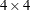unstructured blocks of. There is nomatrix for this model, and each of the 27 subjects has a maximum of 4 observations.

Output 65.2.4: Repeated Measures Analysis (continued)

Number of Observations
Number of Observations Read 108
Number of Observations Used 108
Number of Observations Not Used 0

Iteration History
Iteration Evaluations -2 Log Like Criterion
0 1 478.24175986
1 2 419.47721707 0.00000152
2 1 419.47704812 0.00000000

 Convergence criteria met.

Three Newton-Raphson iterations are required to find the maximum likelihood estimates (Output 65.2.4). The default relative Hessian criterion has a final value less than 1E–8, indicating the convergence of the Newton-Raphson algorithm and the attainment of an optimum.

Output 65.2.5: Repeated Measures Analysis (continued)

Estimated R Matrix for Person 1
Row Col1 Col2 Col3 Col4
1 5.1192 2.4409 3.6105 2.5222
2 2.4409 3.9279 2.7175 3.0624
3 3.6105 2.7175 5.9798 3.8235
4 2.5222 3.0624 3.8235 4.6180

The 44 matrix in Output 65.2.5 is the estimated unstructured covariance matrix. It is the estimate of the first block of, and the other 26 blocks all have the same estimate.

Output 65.2.6: Repeated Measures Analysis (continued)

Covariance Parameter Estimates
Cov Parm Subject Estimate Standard
Error
Z Value Pr Z
UN(1,1) Person 5.1192 1.4169 3.61 0.0002
UN(2,1) Person 2.4409 0.9835 2.48 0.0131
UN(2,2) Person 3.9279 1.0824 3.63 0.0001
UN(3,1) Person 3.6105 1.2767 2.83 0.0047
UN(3,2) Person 2.7175 1.0740 2.53 0.0114
UN(3,3) Person 5.9798 1.6279 3.67 0.0001
UN(4,1) Person 2.5222 1.0649 2.37 0.0179
UN(4,2) Person 3.0624 1.0135 3.02 0.0025
UN(4,3) Person 3.8235 1.2508 3.06 0.0022
UN(4,4) Person 4.6180 1.2573 3.67 0.0001

The "Covariance Parameter Estimates" table in Output 65.2.6 lists the 10 estimated covariance parameters in order; note their correspondence to the first block ofdisplayed in Output 65.2.5. The parameter estimates are labeled according to their location in the block in the Cov Parm column, and all of these estimates are associated with Person as the subject effect. The Std Error column lists approximate standard errors of the covariance parameters obtained from the inverse Hessian matrix. These standard errors lead to approximate Wald Z statistics, which are compared with the standard normal distribution The results of these tests indicate that all the parameters are significantly different from 0; however, the Wald test can be unreliable in small samples.

To carry out Wald tests of various linear combinations of these parameters, use the following procedure. First, run the statements again, adding the ASYCOV option and an ODS statement:

ods output CovParms=cp AsyCov=asy;
proc mixed data=pr method=ml covtest asycov;
class Person Gender;
model y = Gender Age Gender*Age / s;
repeated / type=un subject=Person r;
run;


This creates two data sets, cp and asy, which contain the covariance parameter estimates and their asymptotic variance covariance matrix, respectively. Then read these data sets into the SAS/IML matrix programming language as follows:

proc iml;
use cp;
read all var {Estimate} into est;
use asy;
read all var ('CovP1':'CovP10') into asy;
quit;


You can then construct your desired linear combinations and corresponding quadratic forms with the asy matrix.

Output 65.2.7: Repeated Measures Analysis (continued)

Fit Statistics
-2 Log Likelihood 419.5
AIC (Smaller is Better) 447.5
AICC (Smaller is Better) 452.0
BIC (Smaller is Better) 465.6

Null Model Likelihood Ratio Test
DF Chi-Square Pr > ChiSq
9 58.76 <.0001

The null model likelihood ratio test (LRT) in Output 65.2.7 is highly significant for this model, indicating that the unstructured covariance matrix is preferred to the diagonal matrix of the ordinary least squares null model. The degrees of freedom for this test is 9, which is the difference between 10 and the 1 parameter for the null model’s diagonal matrix.

Output 65.2.8: Repeated Measures Analysis (continued)

Solution for Fixed Effects
Effect Gender Estimate Standard
Error
DF t Value Pr > |t|
Intercept   15.8423 0.9356 25 16.93 <.0001
Gender F 1.5831 1.4658 25 1.08 0.2904
Gender M 0 . . . .
Age   0.8268 0.07911 25 10.45 <.0001
Age*Gender F -0.3504 0.1239 25 -2.83 0.0091
Age*Gender M 0 . . . .

The "Solution for Fixed Effects" table in Output 65.2.8 lists the solution vector for the fixed effects. The estimate of the boys’ intercept is 15.8423, while that for the girls is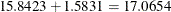. Similarly, the estimate for the boys’ slope is 0.8268, while that for the girls is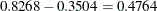. Thus the girls’ starting point is larger than that for the boys, but their growth rate is about half that of the boys.

Note that two of the estimates equal 0; this is a result of the overparameterized model used by PROC MIXED. You can obtain a full-rank parameterization by using the following MODEL statement:

model y = Gender Gender*Age / noint s;


Here, the NOINT option causes the different intercepts to be fit directly as the two levels of Gender. However, this alternative specification results in different tests for these effects.

Output 65.2.9: Repeated Measures Analysis (continued)

Type 3 Tests of Fixed Effects
Effect Num DF Den DF F Value Pr > F
Gender 1 25 1.17 0.2904
Age 1 25 110.54 <.0001
Age*Gender 1 25 7.99 0.0091

The "Type 3 Tests of Fixed Effects" table in Output 65.2.9 displays Type 3 tests for all of the fixed effects. These tests are partial in the sense that they account for all of the other fixed effects in the model. In addition, you can use the HTYPE= option in the MODEL statement to obtain Type 1 (sequential) or Type 2 (also partial) tests of effects.

It is usually best to consider higher-order terms first, and in this case the Age*Gender test reveals a difference between the slopes that is statistically significant at the 1% level. Note that the p-value for this test (0.0091) is the same as the p-value in the "Age*Gender F" row in the "Solution for Fixed Effects" table (Output 65.2.8) and that the F statistic (7.99) is the square of the t statistic (–2.83), ignoring rounding error. Similar connections are evident among the other rows in these two tables.

The Age test is one for an overall growth curve accounting for possible heterogeneous slopes, and it is highly significant. Finally, the Gender row tests the null hypothesis of a common intercept, and this hypothesis cannot be rejected from these data.

As an alternative to the F tests shown here, you can carry out likelihood ratio tests of various hypotheses by fitting the reduced models, subtracting –2 log likelihoods, and comparing the resulting statistics withdistributions.

Since the different levels of the repeated effect represent different years, it is natural to try fitting a time series model to the data within each subject. To obtain time series structures in, you can replace TYPE=UN with TYPE=AR(1) or TYPE=TOEP to obtain the first- or nth-order autoregressive covariance matrices, respectively. For example, the statements to fit an AR(1) structure are as follows:

/* first-order autoregressive */
proc mixed data=pr method=ml;
class Person Gender;
model y = Gender Age Gender*Age / s;
repeated / type=ar(1) sub=Person r;
run;


To fit a random coefficients model, use the following statements:

/* random coefficients model */
proc mixed data=pr method=ml;
class Person Gender;
model y = Gender Age Gender*Age / s;
random intercept Age / type=un sub=Person g;
run;


This specifies an unstructured covariance matrix for the random intercept and slope. In mixed model notation,is block diagonal with identical 22 unstructured blocks for each person. By default,becomes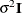. See Example 65.5 for further information about this model.

Finally, you can fit a compound symmetry structure by using TYPE=CS , as follows:

proc mixed data=pr method=ml covtest;
class Person Gender;
model y = Gender Age Gender*Age / s;
repeated / type=cs subject=Person r;
run;


The results from this analysis are shown in Output 65.2.10Output 65.2.17.

The "Model Information" table in Output 65.2.10 is the same as before except for the change in "Covariance Structure."

Output 65.2.10: Repeated Measures Analysis with Compound Symmetry Structure

The Mixed Procedure

Model Information
Data Set WORK.PR
Dependent Variable y
Covariance Structure Compound Symmetry
Subject Effect Person
Estimation Method ML
Residual Variance Method Profile
Fixed Effects SE Method Model-Based
Degrees of Freedom Method Between-Within

The "Dimensions" table in Output 65.2.11 shows that there are only two covariance parameters in the compound symmetry model; this covariance structure has common variance and common covariance.

Output 65.2.11: Analysis with Compound Symmetry (continued)

Class Level Information
Class Levels Values
Person 27 1 2 3 4 5 6 7 8 9 10 11 12 13 14 15 16 17 18 19 20 21 22 23 24 25 26 27
Gender 2 F M

Dimensions
Covariance Parameters 2
Columns in X 6
Columns in Z 0
Subjects 27
Max Obs per Subject 4

Number of Observations
Number of Observations Read 108
Number of Observations Used 108
Number of Observations Not Used 0

Since the data are balanced, only one step is required to find the estimates (Output 65.2.12).

Output 65.2.12: Analysis with Compound Symmetry (continued)

Iteration History
Iteration Evaluations -2 Log Like Criterion
0 1 478.24175986
1 1 428.63905802 0.00000000

 Convergence criteria met.

Output 65.2.13 displays the estimatedmatrix for the first subject. Note the compound symmetry structure here, which consists of a common covariance with a diagonal enhancement.

Output 65.2.13: Analysis with Compound Symmetry (continued)

Estimated R Matrix for Person 1
Row Col1 Col2 Col3 Col4
1 4.9052 3.0306 3.0306 3.0306
2 3.0306 4.9052 3.0306 3.0306
3 3.0306 3.0306 4.9052 3.0306
4 3.0306 3.0306 3.0306 4.9052

The common covariance is estimated to be 3.0306, as listed in the CS row of the "Covariance Parameter Estimates" table in Output 65.2.14, and the residual variance is estimated to be 1.8746, as listed in the Residual row. You can use these two numbers to estimate the intraclass correlation coefficient (ICC) for this model. Here, the ICC estimate equals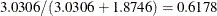. You can also obtain this number by adding the RCORR option to the REPEATED statement.

Output 65.2.14: Analysis with Compound Symmetry (continued)

Covariance Parameter Estimates
Cov Parm Subject Estimate Standard
Error
Z Value Pr Z
CS Person 3.0306 0.9552 3.17 0.0015
Residual   1.8746 0.2946 6.36 <.0001

In the case shown in Output 65.2.15, the null model LRT has only one degree of freedom, corresponding to the common covariance parameter. The test indicates that modeling this extra covariance is superior to fitting the simple null model.

Output 65.2.15: Analysis with Compound Symmetry (continued)

Fit Statistics
-2 Log Likelihood 428.6
AIC (Smaller is Better) 440.6
AICC (Smaller is Better) 441.5
BIC (Smaller is Better) 448.4

Null Model Likelihood Ratio Test
DF Chi-Square Pr > ChiSq
1 49.60 <.0001

Note that the fixed-effects estimates and their standard errors (Output 65.2.16) are not very different from those in the preceding unstructured example (Output 65.2.8).

Output 65.2.16: Analysis with Compound Symmetry (continued)

Solution for Fixed Effects
Effect Gender Estimate Standard
Error
DF t Value Pr > |t|
Intercept   16.3406 0.9631 25 16.97 <.0001
Gender F 1.0321 1.5089 25 0.68 0.5003
Gender M 0 . . . .
Age   0.7844 0.07654 79 10.25 <.0001
Age*Gender F -0.3048 0.1199 79 -2.54 0.0130
Age*Gender M 0 . . . .

The F tests shown in Output 65.2.17 are also similar to those from the preceding unstructured example (Output 65.2.9). Again, the slopes are significantly different but the intercepts are not.

Output 65.2.17: Analysis with Compound Symmetry (continued)

Type 3 Tests of Fixed Effects
Effect Num DF Den DF F Value Pr > F
Gender 1 25 0.47 0.5003
Age 1 79 111.10 <.0001
Age*Gender 1 79 6.46 0.0130

You can fit the same compound symmetry model with the following specification by using the RANDOM statement:

proc mixed data=pr method=ml;
class Person Gender;
model y = Gender Age Gender*Age / s;
random Person;
run;


Compound symmetry is the structure that Jennrich and Schluchter deemed best among the ones they fit. To carry the analysis one step further, you can use the GROUP= option as follows to specify heterogeneity of this structure across girls and boys:

proc mixed data=pr method=ml;
class Person Gender;
model y = Gender Age Gender*Age / s;
repeated / type=cs subject=Person group=Gender;
run;


The results from this analysis are shown in Output 65.2.18Output 65.2.24. Note that in Output 65.2.18 Gender is listed as a "Group Effect."

Output 65.2.18: Repeated Measures Analysis with Heterogeneous Structures

The Mixed Procedure

Model Information
Data Set WORK.PR
Dependent Variable y
Covariance Structure Compound Symmetry
Subject Effect Person
Group Effect Gender
Estimation Method ML
Residual Variance Method None
Fixed Effects SE Method Model-Based
Degrees of Freedom Method Between-Within

The four covariance parameters listed in Output 65.2.19 result from the two compound symmetry structures corresponding to the two levels of Gender.

Output 65.2.19: Analysis with Heterogeneous Structures (continued)

Class Level Information
Class Levels Values
Person 27 1 2 3 4 5 6 7 8 9 10 11 12 13 14 15 16 17 18 19 20 21 22 23 24 25 26 27
Gender 2 F M

Dimensions
Covariance Parameters 4
Columns in X 6
Columns in Z 0
Subjects 27
Max Obs per Subject 4

Number of Observations
Number of Observations Read 108
Number of Observations Used 108
Number of Observations Not Used 0

As Output 65.2.20 shows, even with the heterogeneity, only one iteration is required for convergence.

Output 65.2.20: Analysis with Heterogeneous Structures (continued)

Iteration History
Iteration Evaluations -2 Log Like Criterion
0 1 478.24175986
1 1 408.81297228 0.00000000

 Convergence criteria met.

The "Covariance Parameter Estimates" table in Output 65.2.21 lists the heterogeneous estimates. Note that both the common covariance and the diagonal enhancement differ between girls and boys.

Output 65.2.21: Analysis with Heterogeneous Structures (continued)

Covariance Parameter Estimates
Cov Parm Subject Group Estimate
Variance Person Gender F 0.5900
CS Person Gender F 3.8804
Variance Person Gender M 2.7577
CS Person Gender M 2.4463

As Output 65.2.22 shows, both Akaike’s information criterion (424.8) and Schwarz’s Bayesian information criterion (435.2) are smaller for this model than for the homogeneous compound symmetry model (440.6 and 448.4, respectively). This indicates that the heterogeneous model is more appropriate. To construct the likelihood ratio test between the two models, subtract the –2 log likelihood values:. Comparing this value with thedistribution with two degrees of freedom yields a p-value less than 0.0001, again favoring the heterogeneous model.

Output 65.2.22: Analysis with Heterogeneous Structures (continued)

Fit Statistics
-2 Log Likelihood 408.8
AIC (Smaller is Better) 424.8
AICC (Smaller is Better) 426.3
BIC (Smaller is Better) 435.2

Null Model Likelihood Ratio Test
DF Chi-Square Pr > ChiSq
3 69.43 <.0001

Note that the fixed-effects estimates shown in Output 65.2.23 are the same as in the homogeneous case, but the standard errors are different.

Output 65.2.23: Analysis with Heterogeneous Structures (continued)

Solution for Fixed Effects
Effect Gender Estimate Standard
Error
DF t Value Pr > |t|
Intercept   16.3406 1.1130 25 14.68 <.0001
Gender F 1.0321 1.3890 25 0.74 0.4644
Gender M 0 . . . .
Age   0.7844 0.09283 79 8.45 <.0001
Age*Gender F -0.3048 0.1063 79 -2.87 0.0053
Age*Gender M 0 . . . .

The fixed-effects tests shown in Output 65.2.24 are similar to those from previous models, although the p-values do change as a result of specifying a different covariance structure. It is important for you to select a reasonable covariance structure in order to obtain valid inferences for your fixed effects.

Output 65.2.24: Analysis with Heterogeneous Structures (continued)

Type 3 Tests of Fixed Effects
Effect Num DF Den DF F Value Pr > F
Gender 1 25 0.55 0.4644
Age 1 79 141.37 <.0001
Age*Gender 1 79 8.22 0.0053